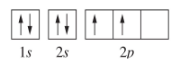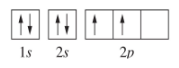# Problem: Write out the four possible quantum numbers for each of the electrons in the diagram below. Give them in the order of: n, l, ml, and ms.HINT: There are 6 electrons therefore you should give 6 answers.

🤓 Based on our data, we think this question is relevant for Professor Blake's class at UCI.

###### FREE Expert Solution

We’re being asked to write the four possible quantum numbers for each of the electrons in the given diagram below:Let’s first define and determine the possible values of the four quantum numbers:

principal quantum number (n)  energy level in orbitals and its value could be any positive integer starting from 1 to infinity

angular momentum quantum number (ℓ) → (l) has to be at least 1 less than n, range of values from 0 up to (n-1)

▪ Each ℓ value corresponds to a subshell:

= 0 → s subshell
= 1 → p subshell
= 2 → d subshell
= 3 → f subshell

• magnetic quantum number (m) → range of values from - to +

▪ The m values (for each ℓ) represent an orbital for the subshell:###### Problem Details

Write out the four possible quantum numbers for each of the electrons in the diagram below. Give them in the order of: n, l, ml, and ms.

HINT: There are 6 electrons therefore you should give 6 answers.What scientific concept do you need to know in order to solve this problem?

Our tutors have indicated that to solve this problem you will need to apply the Quantum Numbers concept. If you need more Quantum Numbers practice, you can also practice Quantum Numbers practice problems.

What is the difficulty of this problem?

Our tutors rated the difficulty ofWrite out the four possible quantum numbers for each of the ...as high difficulty.

How long does this problem take to solve?

Our expert Chemistry tutor, Dasha took 6 minutes and 2 seconds to solve this problem. You can follow their steps in the video explanation above.

What professor is this problem relevant for?

Based on our data, we think this problem is relevant for Professor Blake's class at UCI.